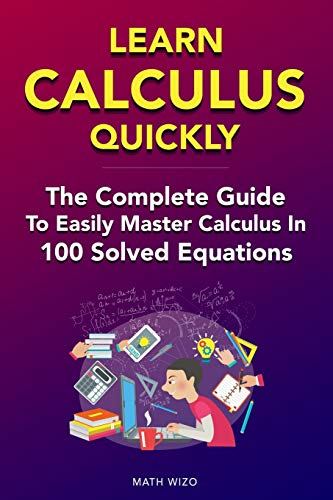Buy it at best price from here

### Book Specification

 Binding Paperback Language English Number Of Pages 86 Author Math Wizo Publisher Independently Published Isbn-10 1796605603 Isbn-13 9781796605600 Dimension 15.24*0.48*22.86

# Learn Calculus Quickly: The Complete Guide to Easily Master Calculus in 100 Solved Equations!

## Math Wizo's Learn Calculus Quickly: The Complete Guide to Easily Master Calculus in 100 Solved Equations!

The Complete Guide To Break Down CalculusThe Calculus study guide contains three Basic sections: Limits DerivativesIntegralsThe Limits section Covers slope and linear equations, tangent lines, the definition of a limit, and evaluating limit functions.The Derivative section Covers differentiation rules for polynomial functions, trigonometric functions, rational functions, exponential functions, radical functions, and the natural logarithmic functions. It also includes the Product Rule, the Quotient Rule, and the Chain Rule, first and second derivatives, and partial derivatives.The Integration section Covers the integration rules for polynomial functions, trigonometric functions, rational functions, exponential functions, radical functions, and the natural logarithmic functions. It has examples of both indefinite and definite integrations.It also includes the Chain Rule, double integrals, and triple integrals. Take Action & Get Yours TodayScroll up and "buy now" so you can learn Calculus once and for all.Simply Order Now!
Amazon, Paperback Rs. 1111.0

## Why you should read Learn Calculus Quickly: The Complete Guide to Easily Master Calculus in 100 Solved Equations! by Math Wizo

This book has been written by Math Wizo, who has written books like Learn Calculus Quickly: The Complete Guide to Easily Master Calculus in 100 Solved Equations!. The books are written in Calculus category. This book is read by people who are interested in reading books in category : Calculus. So, if you want to explore books similar to This book, you must read and buy this book.

## How long would it take for you to read Learn Calculus Quickly: The Complete Guide to Easily Master Calculus in 100 Solved Equations!

#### Depending on your reading style, this is how much time you would take to complete reading this book.

Reading Style Time To Finish The Book 17 hours 8 hours 5 hours 2 hours
So if you are a Reader belonging in the Good category, and you read it daily for 1 hour, it will take you 5 days.
Note: A slow reader usually reads 100 words per minute, an average reader 200 words per minute, an average reader 300 words per minute and an excellent leader reads about 600-1000 words per minute, however the comprehension may vary.

Searches in World for Learn Calculus Quickly: The Complete Guide to Easily Master Calculus in 100 Solved Equations!

 City Country Count 39